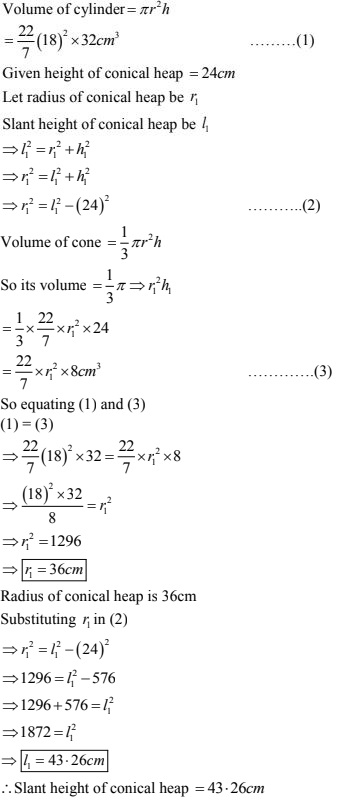# Chapter 16 Surface Areas and Volumes RD Sharma Solutions Exercise 16.1 Class 10 Maths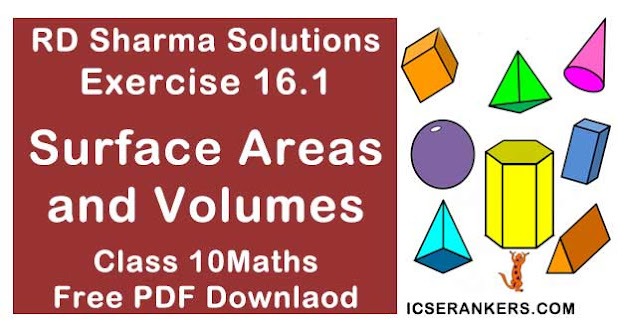Chapter Name RD Sharma Chapter 16 Surface Area and Volumes Exercise 16.1 Book Name RD Sharma Mathematics for Class 10 Other Exercises Exercise 16.2Exercise 16.3 Related Study NCERT Solutions for Class 10 Maths

### Exercise 16.1 Solutions

Solution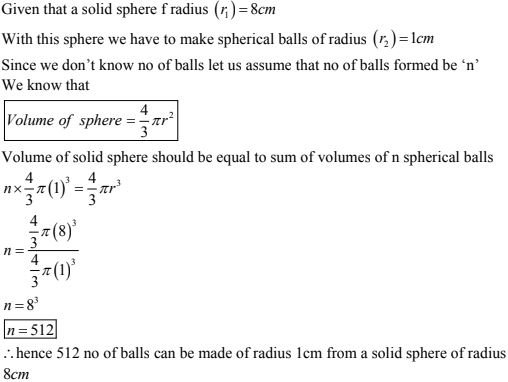2. How many spherical bullets each of 5cm in diameter can be cast from a rectangular block of metal 11dm × 1m × 5dm ?
Solution
Given that a metallic block which is rectangular of diameter 11dm × 1m × 5dm
Given that diameter of each bullet is 5cm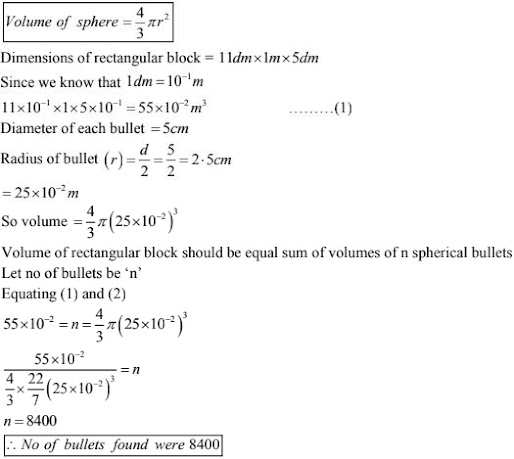3. A spherical ball of radius 3cm is melted and recast into three spherical balls. The radii of the two of balls are 1.9 cm and 2cm. Determine the diameter of the third ball ?
Solution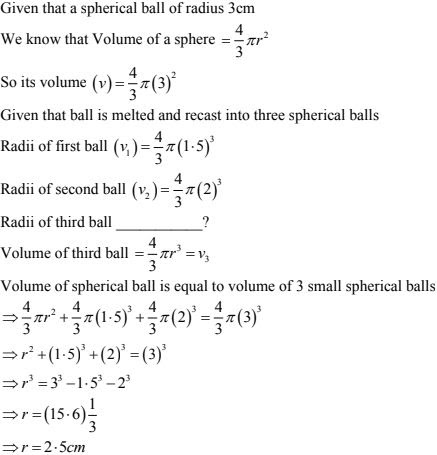Diameter (d) = 2r = 2×2.5 = 5 cm
∴ Diameter of third ball = 5cm.

4. 2.2 cubic dm of grass is to be drawn into a cylinder wire 0.25cm in diameter. Find the length of wire ?
Solution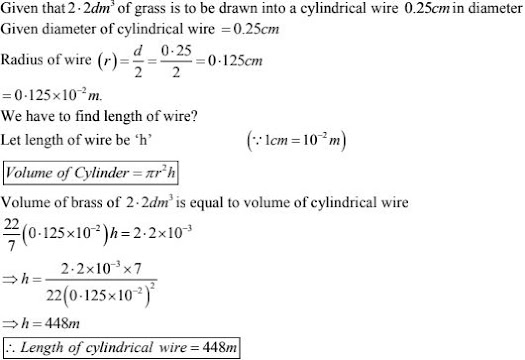5. What length of a solid cylinder 2cm in diameter must be taken to recast into a hollow cylinder of length 16cm, external diameter 20cm and thickness 2.5mm ?
Solution
Given that diameter of solid cylinder = 2cm
Given that solid cylinder is recast to hollow cylinder
Length of hollow cylinder = 16 cm
External diameter = 20 cm
Thickness = 2.5 mm = 0.25cm
Volume of solid cylinder = πr2h
so volume of solid cylinder = π(1)2h
Let length of solid cylinder be h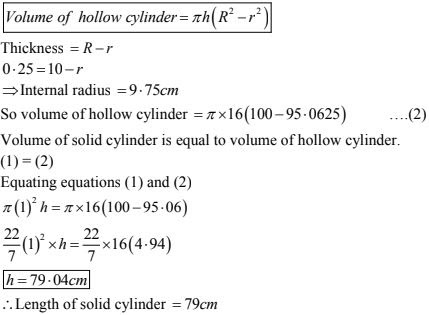6. A cylindrical vessel having diameter equal to its height is full of water which is poured into two identical cylindrical vessels with diameter 42cm and height 21cm which are filled completely. Find the diameter of cylindrical vessel ?
Solution
Given that diameter is equal to height of a cylinder
So h = 2r
Volume of cylinder = πr2h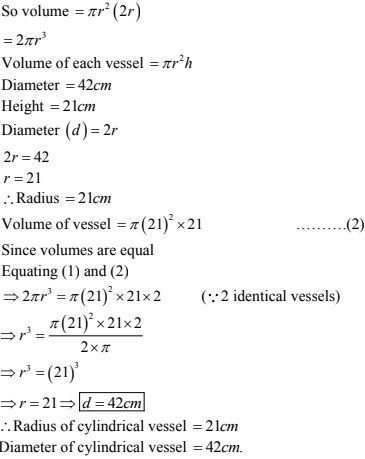7. 50 circular plates each of diameter 14 cm and thickness 0.5cm are placed one above other to form a right circular cylinder. Find its total surface area ?
Solution
Given that 50 circular plates each with diameter = 14cm
Radius of circular plates (r) = 7cm
Thickness of plates = 0.5
Since these plates are placed one above other so total thickness of plates = 0.5 ×50 = 25 cm.
Total surface area of a cylinder = 2πrh + 2πr2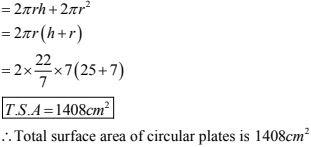8. 25 circular plates each of radius 10.5cm nd thickness 1.6cm are placed one above the other to form a solid circular cylinder. Find the curved surface area and volume of cylinder so formed ?
Solution
Given that 25 circular plates each with radius (r) = 10.5 cm
Thickness = 1.6cm
Since plates are placed one above other so its height becomes = 1.6 × 25 = 40 cm
Volume of cylinder = πr2h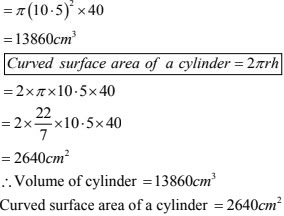9. A path 2m wide surrounds a circular pond of diameter 4cm. How many cubic meters of gravel are required to grave the path to a depth of 20cm .
Solution
Diameter of circular pond = 40m
Radius of pond (r) = 20m.
Thickness = 2m
Depth = 20cm = 0.2m
Since it is viewed as a hollow cylinder
Thickness(t) = R - r
2 = R - r
2 = R - 20
R = 22 m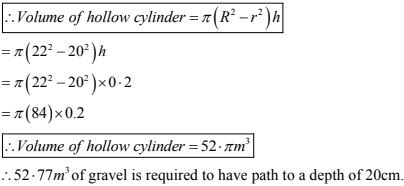10. A  16m deep well with diameter 3.5m is dug up and the earth from it is spread evenly to form a platform 27.5 m by 7m. find height of platform ?
Solution
Let as assume well is a solid right circular cylinder
Radius of cylinder (r) = 3.5/2 = 1.75 m
Height (or) depth of well = 16m.
Volume of right circular cylinder = πr2h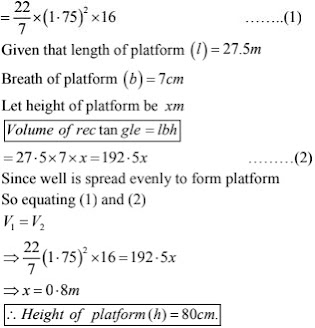11. A well of diameter 2m is dug 14m deep. The earth taken out of it is spread evenly all around it to form an embankment of height 40cm. Find width of the embankment ?
Solution
Let us assume well as a solid circular cylinder
Radius of circular cylinder  = 2/2 = 1 m
Height (or) depth of well = 14m
Volume of solid circular cylinder = πr2h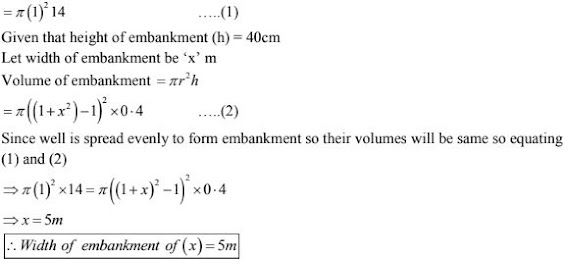12. Find the volume of the largest right circular cone that can be cut out of a cube where edge is 9cm?
Solution
Given that side of cube  = 9cm
Given that largest cone is curved from cube
Diameter of base of cone = side of cube
⇒ 2x = 9
⇒ r = 9/2 cm
Height of cone  = side of cube
⇒ Height of cone(h) = 9cm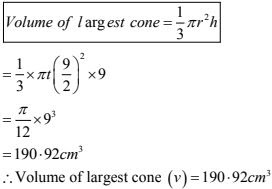13. A cylindrical bucket, 32 cm high and 18cm of radius of the base, is filled with sand. This bucket is emptied on the ground and a conical heap of sand is formed. If the height of the conical heap is 24cm, find the radius and slant height of the heap.
Solution

Given, a cylindrical bucket is filled with sand.

Cylindrical bucket has a height 32 cm and a base radius of 18 cm.

Bucket is emptied on the ground and a conical heap of sand is formed.

Height of the conical heap of sand is 24 cm

We have to find the radius and slant height of the heap.

Volume of cylindrical bucket = πr²h

Given, r = 18 cm

h = 32 cm

Volume of sand in cylindrical bucket = (22/7)(18)²(32)

= 32585.14 cm³

Volume of cone = (1/3)πr²h

Given, h = 24 cm

= (1/3)(22/7)r²(24)

= 25.14r² cm³

Given, volume of sand in a cylindrical bucket = volume of conical heap of sand.

25.14r² = 32585.14

r² = 32585.14/25.14

r² = 1296.14

Taking square root,

r = √1296.14

r = 36 cm

Therefore, the radius of the conical heap of sand is 36 cm.

Slant height, l = √r² + h²

= √(36)² + (24)²

= √1296 + 576

= √1872

= 43.27 cm

Therefore, the slant height of the conical heap of sand is 43.27 cm.

14. Rain water, which falls on a flat rectangular surface of length 6cm and breath 4m is transferred into a cylindrical vessel of internal radius 10cm. What will be the height of water in the cylindrical vessel if a rainfall of 1cm has fallen?
Solution
Given length of rectangular surface = 6cm
Breath of rectangular surface = 4cm
Height (h) = 1cm
Volume of a flat rectangular surface = lbh
6000 × 400 × l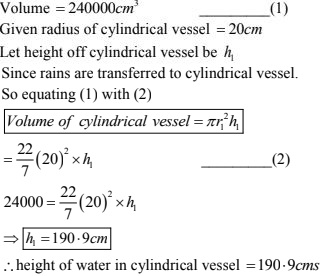15. A conical flask is full of water. The flask has base radius r and height h. The water is proved into a cylindrical flask off base radius one. Find the height of water in the cylindrical flask ?
Solution
Height of conical flask is h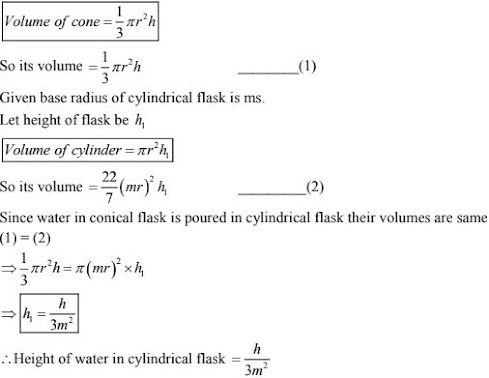16. A rectangular tank 15m long and 11m broad is required to receive entire liquid contents from a full cylindrical tank of internal diameter 21m and length 5m. Find least height of tank that will serve purpose?
Solution
Given length of rectangular tank = 15m
Breath of rectangular tank = 11m
Let height of rectangular tank be h
Volume of rectangular tan k = lbh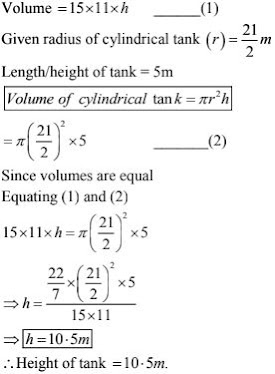17. A hemisphere tool of internal radius 9cm is full of liquid. This liquid is to be filled into cylindrical shaped small bottles each of diameter 3cm and height 4cm. How many bottles are necessary to empty the bowl.
Solution
Given that internal radius of hemisphere bowl = 90m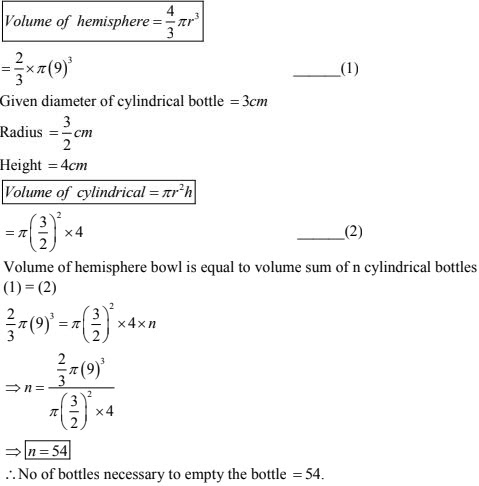18. The diameters of the internal and external surfaces of a hollow spherical shell are 6cm and 10cm respectively. If it is melted and recast and recast into a solid cylinder of diameter 14cm, find the height of the cylinder.
Solution
Internal diameter of hollow spherical shell = 6cm
Internal radius of hollow spherical shell = 6/2 = 3cm
External diameter of hollow spherical shell = 10cm
External radius of hollow spherical shell = 10/2 = 5cm
Diameter of cylinder = 14cm
Radius of cylinder = 14/2 = 7cm
Let height of cylinder = x cm
According to the question
Volume of cylinder = Volume of spherical shell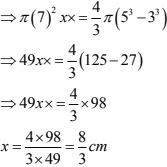∴Height off cylinder = 8/3 cm

19.A hollow sphere of internal and external diameter 4cm and 8cm is melted into a cone of base diameter 8 cm. Calculate height of cone ?
Solution
Given internal diameter of hollow sphere (r) = 4cm
External diameter (R) = 8cm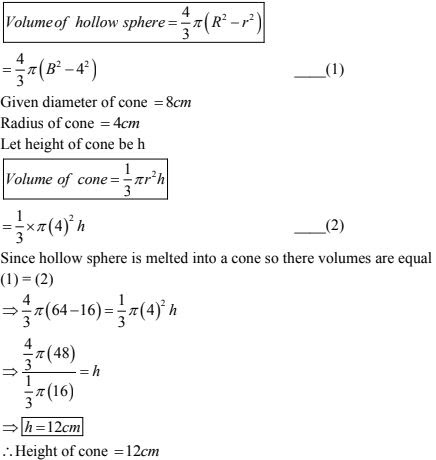20. A cylindrical tube or radius 12cm contains water to a depth of 20cm. A spherical ball is dropped into the tube and the level of the water is raised by 6.75cm. Find the radius of the ball ?
Solution
Given that radius of a cylindrical tube (r) = 12cm
Level of water raised in tube (h) = 6.75cm
Volume of cylinder = πr2h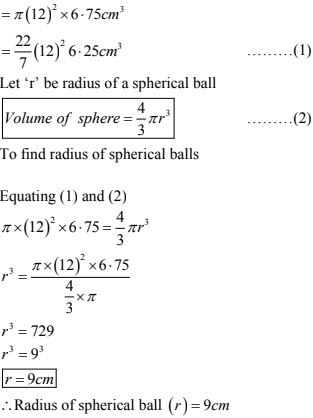21. 500 persons have to dip in a rectangular tank which is 80m long and 50m broad. What is the rise in the level of water in the tank, if the average displacement of water by a person is 0.04m3 ?
Solution
Given that length of a rectangular tank (r) = 80m
Breath of a rectangular tank (b) = 50m
Total displacement of water in rectangular tank
By 500 persons = 500 × 0.04 m3
= 20m3 ...(1)
Let depth of rectangular tank be h
Volume of rectangular tan k = lbh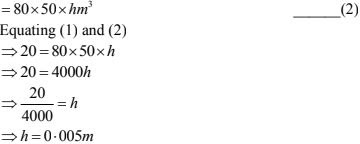h = 0.5cm
∴ Rise in level of water in tank (h) = 0.05cm.

22. A cylindrical jar of radius 6cm contains oil. Iron sphere each of radius 1.5cm are immersed in the oil. How many spheres are necessary to raise level of the oil by two centimetres?
Solution
Given that radius of a cylindrical jar (r) = 6cm
Depth/height of cylindrical jar (h) = 2cm
Let no of balls be 'n'
Volume of a cylinder =  πr2h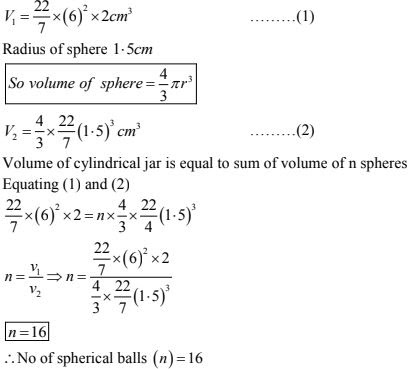23. A hollow sphere of internal and external radii 2cm and 4cm is melted into a cone of base radius 4cm. Find the height and slant height of the cone?
Solution
Given that internal radii of hollow sphere(r) = 2cm
External radii of hollow sphere (R) = 4cm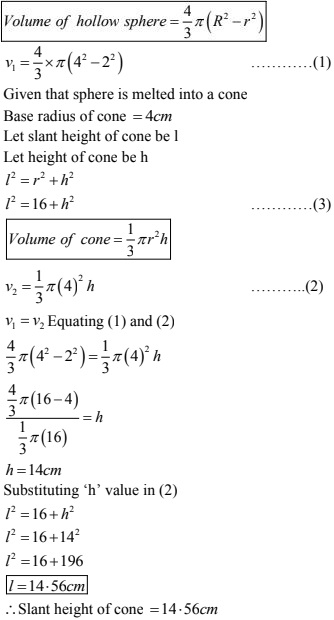24. The internal and external diameters of a hollow hemisphere vessel are 21cm and 25.2cm. The cost of painting 1cm2 of the surface is 10 paise. Find total cost to paint the vessel all over?
Solution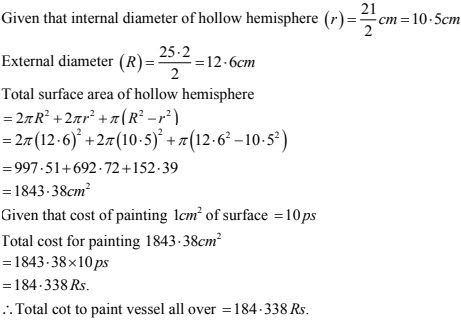25. A cylindrical tube of radius 12cm contains water to a depth of 20cm. A spherical ball of radius 9cm is dropped into the tube and thus level of water is raised by h cm. What is the value of h?
Solution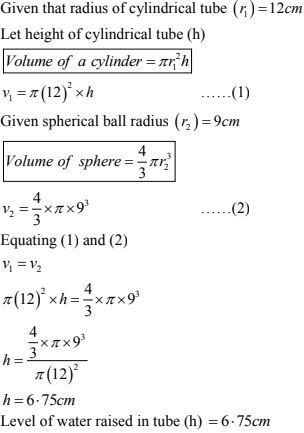26. The difference between outer and inner curved surface areas of a hollow right circular cylinder 14cm long is 88cm2. If the volume of metal used in making cylinder is 176 cm2. Find the outer and inner diameters of the cylinder?
Solution
Given height of a hollow cylinder = 14 cm
Let internal and external radii of hollow
Cylinder be 'r' and R
Given that difference between inner and outer
Curved surface = 88cm2
Curved surface area of cylinder (hollow)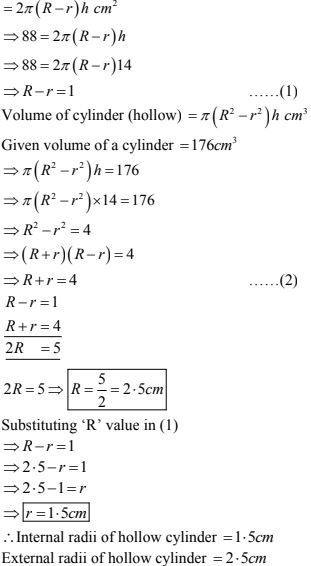27. Prove that the surface area of a sphere is equal to the curved surface area of the circumference cylinder?
Solution
Let radius of a sphere be r
Curved surface area of sphere = 4πr2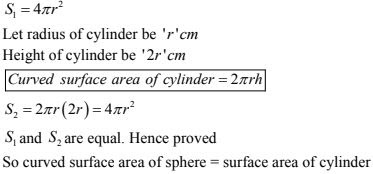28. The diameter of a metallic sphere is equal to 9cm. It is melted and drawn into a long wire of diameter 2mm having uniform cross-section. Find the length of the wire ?
Solution
Given diameter of a sphere (d) = 9cm
Radius (r) = 9/2 = 4.5cm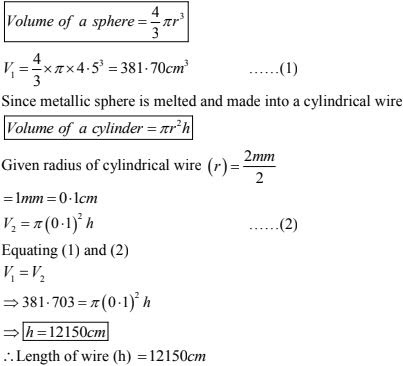29. An iron spherical ball has been melted and recast into smaller balls of equal size. If the radius of each of the smaller balls is 1/4 of the radius of the original ball, how many such balls are made ? Compare the surface area, of all the smaller balls combined together with that of the original ball.
Solution
Given that radius of each of smaller ball = 1/4 Radius of original ball.
Let radius of smaller ball be r.
Radius of bigger ball be 4r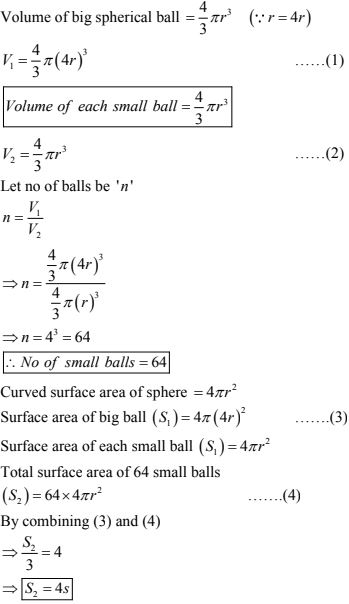∴ Total surface area of small balls is equal to 4 times surface area of big ball.

30. A tent of height 77 dm is in the form a right circular cylinder of diameter 36m and height 44dm surmounted by a right circular cone. Find the cost of canvas at Rs. 3.50 per m2 ?

Solution
Given that height of a tent = 77dm
Height of cone = 44 dm
Height of a tent without cone = 77 - 44 = 33dm = 3.3m
Given diameter of cylinder(d) = 36m
Let 'l' be slant height of cone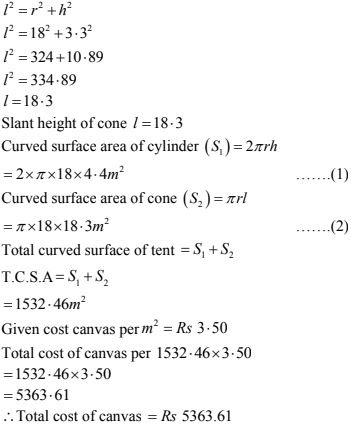31. Metal spheres each of radius 2cm are packed into a rectangular box of internal dimension 16cm × 8cm × 8cm when 16 spheres are packed the box is filled with preservative liquid. Find volume of this liquid ?
Solution
Given radius of metal spheres = 2cm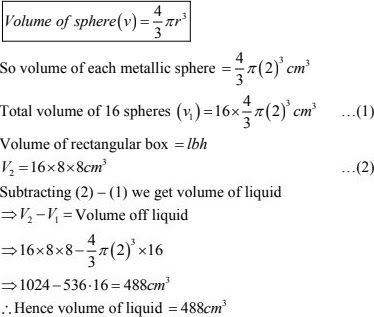32. The largest sphere is to be curved out of a right circular of radius 7cm and height 14cm. Find volume of sphere ?
Solution
Given radius of cylinder (r) = 7cm
Height of cylinder (h) = 14cm
Largest sphere is curved out from cylinder
Thus diameter of sphere = diameter of cylinder
Diameter of sphere (d) = 2 × 7 = 14cm33. A copper sphere of radius 3cm is melted and recast into a right circular cone of height 3cm. Find radius of base of cone ?
Solution
Given radius of sphere = 3cm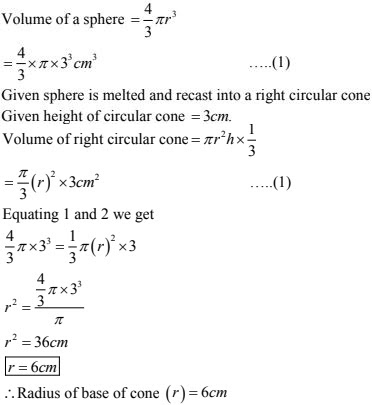34. A vessel in the shape of cuboid contains some water. If these identical spheres are immersed in the water, the level of water is increased by 2cm. If the area of base of cuboid is 160cm2 and its height 12cm, determine radius of any of spheres ?
Solution
Given that area of cuboid = 160cm2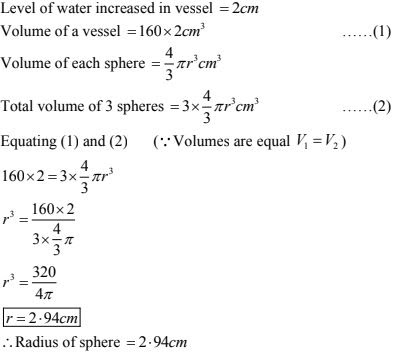35. A copper rod of diameter 1 cm and length 8cm is drawn into a wire of length 18m of  uniform thickness. Find thickness of wire ?
Solution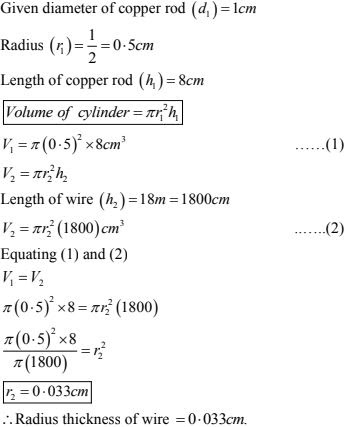36. The diameters of internal and external surfaces of hollow spherical shell are 10 cm and 6 cm respectively. If it is melted and recast into a solid cylinder of length of 2 (2/3)cm, find the diameter of the cylinder.
Solution
Given diameter of internal surfaces of a hollow spherical shell = 10cm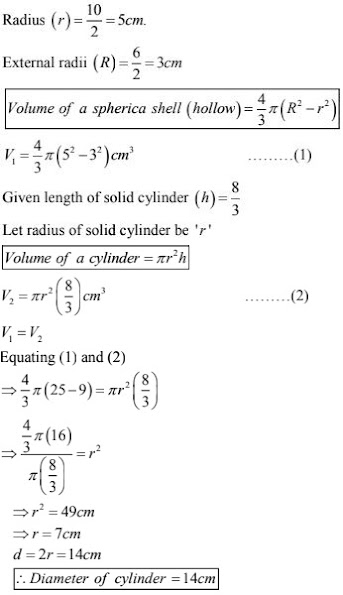37. A right angled triangle whose sides are 3 cm, 4 cm and 5 cm is revolved about the sides
containing the right angle in two days. Find the difference in volumes of the two cones so
formed. Also, find their curved surfaces.
Solution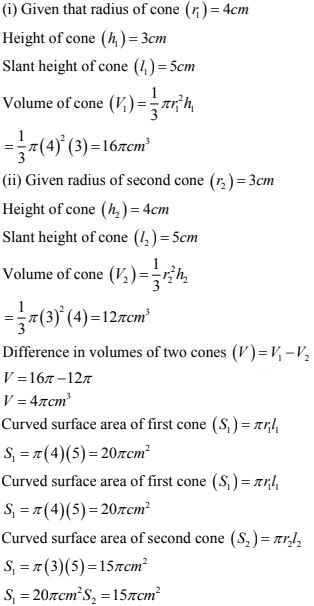38. How many coins 1.75 cm in diameter and 2mm thick must be melted to form a cuboid 11cm × 10cm × 75cm?
Solution
Given that dimensions of a cuboid 11cm × 10cm × 75cm39. A well with inner radius 4 m is dug 14m deep earth taken out of it has been spread evenly
all around a width of 3m it to form an embankment. Find the height of the embankment?
Solution
Given that inner radius of a well (a) = 4m
Depth of a well (h) = 14m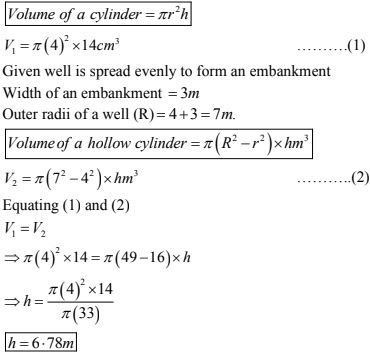40. Water in a canal 1.5m wide and 6m deep is flowering with a speed of 10 km/hr . How much area will it irrigate in 30 minutes if 8cm of standing water is desired?

Solution

Given that water is flowering with a speed = 10 km/hr
In  30 minutes length of flowering standing water = 10× 30/60 km
= 5 km = 5000 m.
Volume of flowering water in 30 minutes
V = 5000 × width × depth m3
Given width of canal = 1.5m
Depth of canal = 6m
V = 5000 × 1.5 ×6 m3
V = 45000 m3
Irrigating area in 30 minutes if 8cm of standing water is desired = 45000/0.08
= 45000/0.08 = 562500m2
∴ Irrigated area in 30 minutes = 562500 m2

41. A farmer runs a pipe of internal diameter 20 cm from the canal into a cylindrical tank in his field which is 10 m in diameter and 2 m deep. If water flows through the pipe at the rate of
3 km/h, in how much time will the tank be filled?

Solution

Volume of pipe = Volume of tank
⇒ πr2h = πR2H
⇒ (10/100)2 × h = (5)2 × 2
⇒ h/100 = 50
⇒ h = 5000m = 5km

Speed of water = 3km/hr
Time taken to fill tank = 5/3 hr = 5/3 × 60min = 100 min

42. A well of diameter 3 m is dug 14 m deep. The earth taken out of it has been spread evenly
all around it to a width of 4 m to form an embankment. Find the height of the embankment.
Solution
Given diameter of well = 3m
Radius of well = 3/2 m = 4
Depth of well(b) = 14m
With of embankment  = 4m
∴ Radius of outer surface of embankment  = 4 + 3/2 = 11/2  m
Let height of embankment  = hm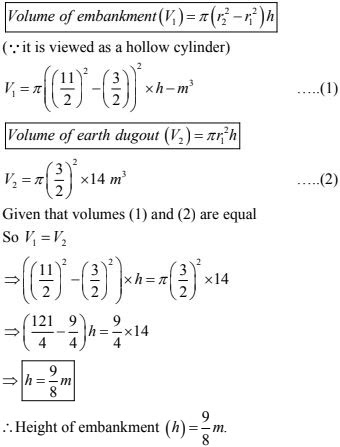43. The surface area of a solid metallic sphere is 616 cm2 . It is melted and recast into a cone of height 28 cm. Find the diameter of the base of the cone so formed (Use it 22/7)
Solution
Given height of cone (h) = 28cm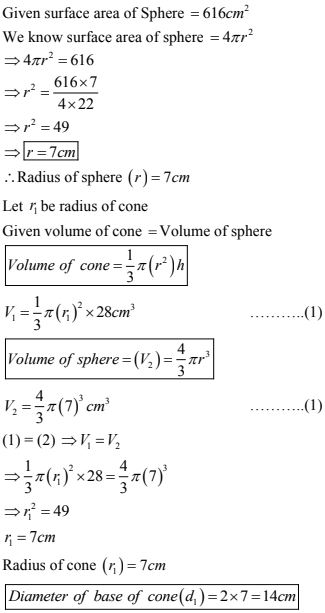44. The difference between the outer and inner curved surface areas of a hollow right circular cylinder 14cm long is 88cm2 . If the volume of metal used in making cylinder is  176 cm3 find outer and inner diameters of the cylinder ?
Solution
Given height of a hollow cylinder = 14cm
Let internal and external radii of hollow
Cylinder be 'r' and 'R'
Given that difference between inner and outer curved surface = 88 cm2 .
Curved surface area of hollow cylinder = 2π(R - r)h
⇒ 88 = 2π(R - 0)h
⇒ 88 = 2π(R - r)14
⇒ R - r = 1 ...(1)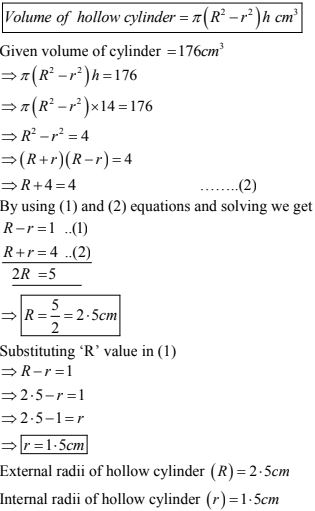45. The volume of a hemisphere is  2425 (1/2)cm3 . Find its curved surface area ?
Solution
Given that volume of a hemisphere = 2424 (1/2)cm3 .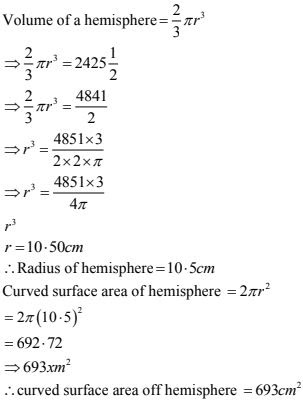46. A cylindrical bucket 32 cm high and with radius of base 18 cm is filled with sand. This bucket is emptied out on the ground and a conical heap of sand is formed. If the height of the conical heap of sand is formed. If the height of the conical heap is 24 cm. find the radius and slant height of the heap ?
Solution
Given that height of cylindrical bucket (h) = 32 cm